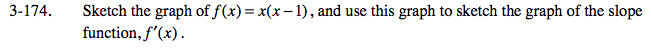### Home > CALC > Chapter 3 > Lesson 3.4.3 > Problem3-174

3-174.f(x) is a parabola,so f '(x) will be linear.

Notice the negative slope when x < 0.5. This means the graph of f '(x) will have negative values on this domain.

Notice the positive slope when x > 0.5. This means the graph of f '(x) will have positive values on this domain.

Notice that x = 0.5 is the x-value of the local minimum. This means the graph of f '(x) will have a root at x = 0.5.

Use the eTool below to visualize this problem.
Click the link at right for the full version of the eTool: Calc 3-174 HW eTool